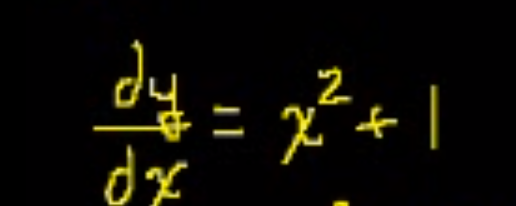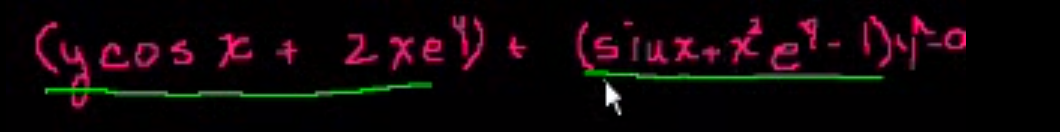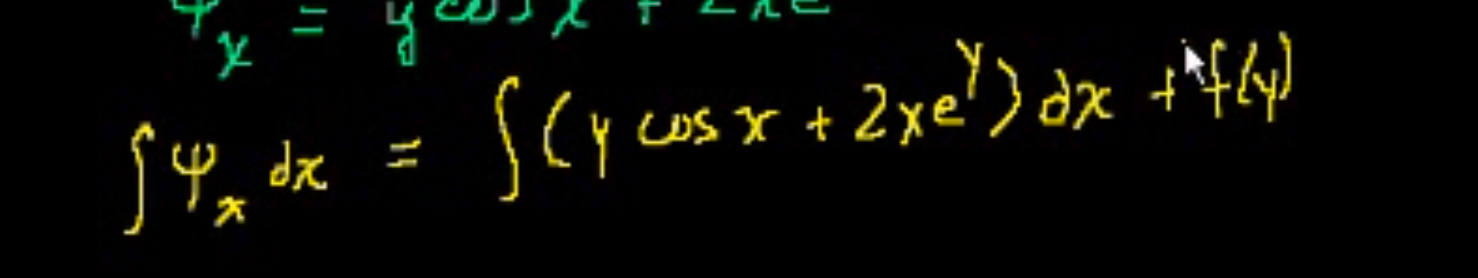Studying for the first stupid midterm in a stupid class. Looks like I broke out the old Khan Academy though, nice. Used to watch a ton of the biology ones for fun when I was in high school.

```For simple differentials, which don't really have y represented, such asWe can just multiply by dx, then take the integral of both sides.

We can do the same thing for stuff with y on the right hand side sometimes, too.

For separable equations, we want to separate the dy and dx on either side, so that you have all y's on one side, and all x's on the other side. Then you integrate.

For exact equations (m_y = n_x). Let's say you have an equation that has a function of x and y plus another function of x and y times dy/dx.￼
Then, you may try to make it separable, but it won't be. It's an exact equation. To test if it's an exact equation, we have M(x,y) + N(x,y)dy/dx = 0.

Then you calculate M_y and N_x, and if they're equal, then it's an exact equation.

So then, take Psi_x = M and Psi_y=N, and then integrate one of them with respect to its variable and add f(y) or f(x) if appropriate, then differentiate with respect to the other variable and set it equal to the other and solve for what should now be f'(y) after differentiating. Then you should be able to just plug in f(y) into the equation and you will have something using which you can solve for y.Integrating Factors
Say we have an equation of the form dy/dx + a(t)*y = p(t).

Well, what we can do is multiply the entire equation by μ(t)= e^integral(a(t)). And then we should have it that the left hand side is the result of the product rule, which means we can integrate both sides, and then solve for y.

Homogeneous Equation

If you look at the order of each of the terms, and they all have the same order (the order of xy is 2, as x^1 * y^1), then you know that its a homogeneous equation. It would look like the form M(x,y)dx+N(x,y)dy=0 (so you may think it's separable, but separation won't work).

If the equation can be written of the form dy/dx = F(y/x). Note that you may have to divide the entire equation by x or by x^2 or x^n in order to make it of the form F(y/x)

Then substitute u=y/x, then you say y=ux, and then dy=udx+xdu, and then you can plug that into the original equation, to get things in terms of only u and x. (plug in u=y/x and dy=udx+xdu). Separate them so you have u's on one side and x's on the other, integrate and you're done.

Bernoulli Equation

If you have a y that's a large order, divide by that entire y^n so that that term becomes 1 or something. THen solve as a separable or homogenous.
```